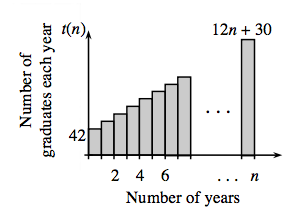### Home > INT3 > Chapter 10 > Lesson 10.2.1 > Problem10-98

10-98.
1.According to the school secretary, the size of the graduating class of Gauss High has steadily increased since the school opened. She claims that the number of graduates each year forms the sequence t(n) = 12n + 30, where n is the number of years the high school has had students graduate. At the end of the year, the administration mails out invitations to all previous graduates to attend the upcoming graduation ceremony. Homework Help ✎

1. In this situation, what does the sequence represent?
What does the series represent?

2.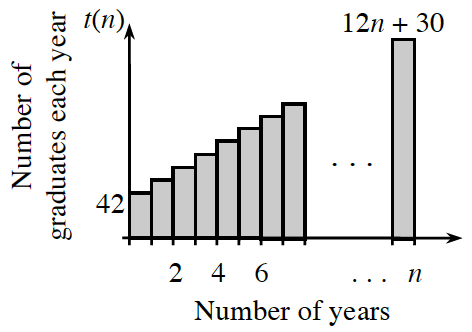After the 10th graduation ceremony, how many total graduates are there? Explain how you determined your answer.

3. The total number of Gauss High graduates after n years can be represented by the graph at right. If the alumni relations committee wants to invite all graduates to a party to celebrate the nth anniversary of the school, how may invitations will they need to send?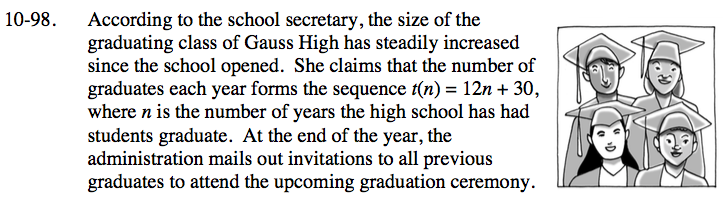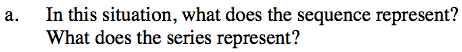Remember that a sequence is a set of discrete numbers while a series is the sum of those numbers.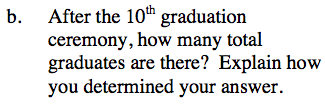$\text{Use the summation formula }S_{10}=\frac{10}{2}\left(t\left(1\right) + t\left(10\right)\right).$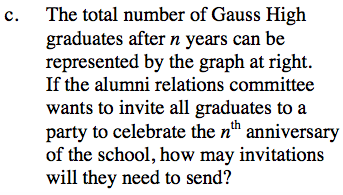See part (b).

n(36 + 6n) = 36n + 6n2